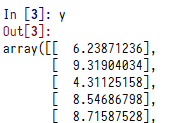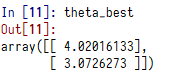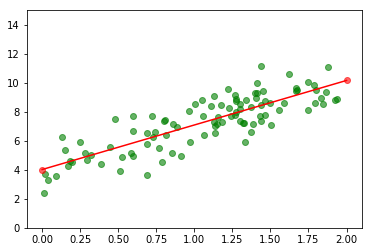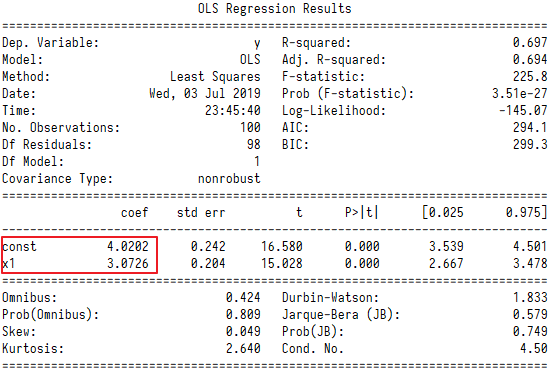# 机器学习实战之训练模型-深入分析线性回归

## 1. 模型说明

$$\hat{y}=θ_0+θ_1x_1+θ_2x_2+…+θ_nx_n$$

• $$\hat{y}$$是预测值
• $$n$$是特征的数量
• $$x_i$$是第i个特征值
• $$θ_j$$是第j个模型参数（包括偏置项$$θ_0$$以及特征权重$$θ_1$$,$$θ_2$$,…,$$θ_n$$）

$$\hat{y} = h_θ(X) = θ^T·X$$

•  $$θ$$是模型的参数向量（列向量）
•  $$θ^T$$是$$θ$$的转置向量（行向量）
•  $$X$$是实例特征向量（矩阵，包括$$x_0$$到$$x_n$$，$$x_0$$永远为1）
•  $$h_θ$$是使用模型参数$$θ$$的假设函数
•  $$θ^T·X$$是$$θ^T$$和$$X$$的点积

## 2. 成本函数

$$MSE=\sum_{i=1}^{n}(y_i-\hat{y}_i)^2$$

$$\hat{θ} = (X^T·X)^{-1}·X^T·y$$

• $$\hat{θ}$$是使得成本函数最小的$$θ$$值
• $$y$$是包含$$y^{(1)}$$到$$y^{(n)}$$的目标值向量（因变量向量）

## 3. 标准方程Python实现

### 3.1 生成线性数据来进行模型拟合

import numpy as np
import matplotlib.pyplot as plt

X = 2 * np.random.rand(100,1)#生产100个1维随机数
y = 4 + 3 * X +np.random.randn(100,1)#生成满足y=4+3x的数据，加入一些随机值### 3.2 标准方程求解

X2 = np.c_[np.ones((100,1)),X]#增加100个1的1维向量和X组合在一起，变成两个特征的向量，请大牛解析下面一行代码
theta_best = np.linalg.inv(X2.T.dot(X2)).dot(X2.T).dot(y)

X2是这样的：X_new = np.array([,]) #x=0和x=2进行预测，X_new为列向量
X_new_b = np.c_[np.ones((2,1)),X_new] #X_new列向量增加一列常量为1的向量
y_predict = X_new_b.dot(theta_best)
y_predictplt.scatter(X,y,c='green', alpha=0.6)
plt.scatter(X_new,y_predict,c='red', alpha=0.6)
plt.plot(X_new,y_predict,c='red')
plt.axis([-0.1,2.1,0,15]) #设置坐标轴的X和Y的最大值和最小值
plt.show()### 3.3 Scikit-Learn实现

from sklearn.linear_model import LinearRegression
reg = LinearRegression()
reg.fit(X,y)#sklearn的x不需要增加一个值为1的特征向量，默认回归是包含截距的
reg.intercept_,reg.coef_
reg.predict(X_new)### 3.4 statsmodels实现

import statsmodels.api as sm
x1 = sm.add_constant(X) #X是一维，通过一个简单的函数，就可以增加一个值为1的特征向量，实现了X2 = np.c_[np.ones((100,1)),X]
models = sm.OLS(y,x1)
rs = models.fit()
print(rs.summary())rs.predict(X_new_b)

array([ 4.02016133, 10.16541594])

### 3.5 梯度下降实现

eta = 0.1
n_iterations =1000
m = 100
theta = np.random.randn(2,1)
for iteration in range(n_iterations):
theta = theta - eta * gradients
theta

#### 请关注我的微信公众号